An analyst was using SAS to analyze some data from an experiment. He noticed that the response variable is always positive (such as volume, size, or weight), but his statistical model predicts some negative responses. He posted the data and asked if it is possible to modify the graph so that only positive responses are displayed.

This article shows how you can truncate a surface or a contour plot so that negative values are not displayed. You could do something similar to truncate unreasonably high values in a surface plot.

### Why does the model predict negative values?

Before showing how to truncate the surface plot, let's figure out why the model predicts negative values when all the observed responses are positive. The following DATA step is a simplified version of the real data. The RSREG procedure uses least squares regression to fit a quadratic response surface. If you use the PLOTS=SURFACE option, the procedure automatically displays a contour plot and surface plot for the predicted response:

```data Sample; input X Y Z @@; datalines; 10 90 22 22 76 13 22 75 7 24 78 14 24 76 10 25 63 5 26 62 10 26 94 20 26 63 15 27 94 16 27 95 14 29 66 7 30 69 8 30 74 8 ;   ods graphics / width=400px height=400px ANTIALIASMAX=10000; proc rsreg data=Sample plots=surface(fill=pred overlaypairs); model Z = Y X; run;   proc rsreg data=Sample plots=surface(3d fill=Pred gridsize=80); model Z = Y X; ods select Surface; ods output Surface=Surface; /* use ODS OUTPUT to save surface data to a data set */ run;```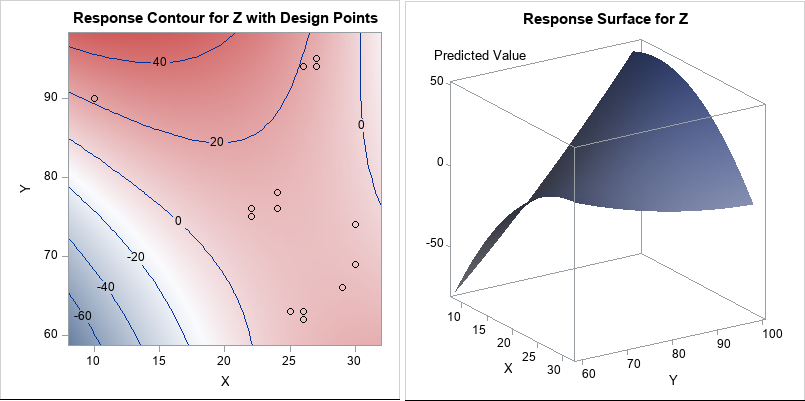The contour plot overlays a scatter plot of the data. You can see that the data are observed only in the upper-right portion of the plot (the red regions) and that no data are in the lower-left portion of the plot. The RSREG procedure fits a quadratic model to the data. The predicted values near the observed data are all positive. Some of the predicted values that are far from the observed data are negative.

I previously wrote about this phenomenon and showed how to compute the convex hull for these bivariate data. When you evaluate the model inside the convex hull, you are interpolating. When you evaluate the model outside the convex hull, you are extrapolating. It is well known that polynomial regression models can give nonsensical results if you extrapolate far from the data.

### Truncating a response surface

The RSREG procedure is not aware that the response variable should be positive. A quadratic surface will eventually get arbitrarily big in the positive and/or negative directions. You can see this on the contour and surface plots, which show the predictions of the model on a regular grid of (X, Y) values.

If you want to display only the positive portion of the prediction surface, you can replace each negative predicted value with a missing value. The first step is to obtain the predicted values on a regular grid. You can use the "missing value trick" to score the quadratic model on a grid, or you can use the ODS OUTPUT statement to obtain the gridded values that are used in the surface plot. I chose the latter option. In the previous section, I used the ODS OUTPUT statement to write the gridded predicted values for the surface plot to a SAS data set named Surface.

As Warren Kuhfeld points out in his article about processing ODS OUTPUT data set, the names in an ODS data object can be "long and hard to type." Therefore, I rename the variables. I also combine the gridded values with the original data so that I can optionally overlay the data and the predicted values.

```/* rename vars and set negative responses to missing */ data Surf2; set Surface(rename=( Predicted0_1_0_0 = Pred /* rename the long ODS names */ Factor1_0_1_0_0 = GY /* 'G' for 'gridded' */ Factor2_0_1_0_0 = GX)) Sample(in=theData); /* combine with original data */ if theData then Type = "Data "; else Type = "Gridded"; if Pred < 0 then Pred = .; /* replace negative predictions with missing values */ label GX = 'X' GY = 'Y'; run;```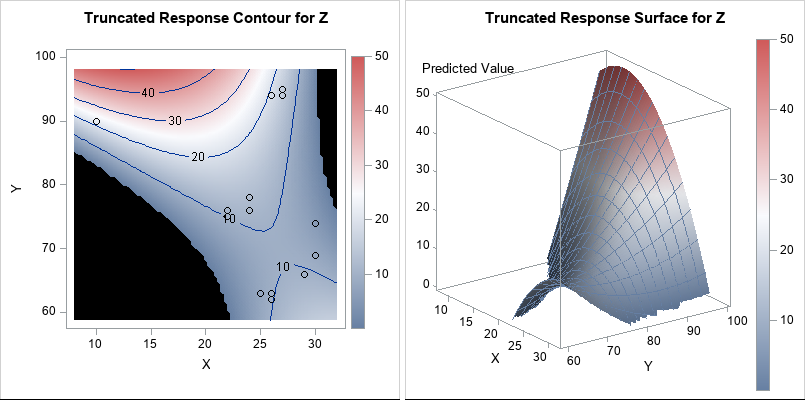You can use the Graph Template Language (GTL) to generate graphs that are similar to those produced by PROC RSREG. You can then use PROC SGRENDER to create the graph. Because the negative response values were set to missing, the contour plot displays a missing value color (black, for this ODS style) in the lower-left and upper-right portions of the plot. Similarly, the missing values cause the surface plot to be truncated. By using the GRIDSIZE= option, you can make the jagged edges small.

Notice that the colors in the graphs are now based on the range [0, 50], whereas previously the colors were based on the range [-60, 50]. I've added a continuous legend to the plots so that the range of the response variable is obvious.

I'd like to stress that sometimes "nonsensical values" indicate an inappropriate model. If you notice nonsensical values, you should always ask yourself why the model is predicting those values. You shouldn't modify the prediction surface without a good reason. But if you do have a good reason, the techniques in this article should help you.

The post Truncate response surfaces appeared first on The DO Loop.A previous article shows how to use a scatter plot to visualize the average SAT scores for all high schools in North Carolina. The schools are grouped by school districts and ranked according to the median value of the schools in the district. For the school districts that have many schools, the markers might overlap, which makes it difficult to visualize the distribution of scores. This is a general problem with using dot plots. An alternative visualization is to plot a box plot for each school district, which is described in today's article.

### Box plots are not for everyone

Box plots (also called box-and-whisker plots) are used by statisticians to provide a schematic visualization of the distribution of some quantity. The previous article was written for non-statisticians, so I did not include any box plots. To understand a box plot, the reader needs to know how to interpret the box and whiskers:

• The lower and upper portion of the box correspond to the 25th and 75th percentiles of the data, respectively
• The line in the middle of the box indicates the median score.
• A diamond marker indicates the mean score.
• The standard box plot extends the whiskers to observations that are within a distance D from the box, where D is 1.5 times the interquartile range. Other kinds of box plots are sometimes used. The SAS documentation explains the parts of a box plot.

Box plots require a certain level of comfort with statistical ideas. Nevertheless, for a statistical audience, box plots provide a compact way to compare dozens or hundreds of distributions.

### The BOXPLOT procedure: An alternative way to display many box plots

I almost always use the SGPLOT procedure to create box plots, but today I'm going to demonstrate the BOXPLOT procedure. The BOXPLOT procedure is from the days before ODS graphics, but it has several nice features, including the following:

1. When grouping box plots into categories, you can add headers, category dividers, and colors to help distinguish the categories. I demonstrated these so-called "nested box plots" in a previous blog post.
2. When you want to display statistics about each box plot as a table inside the graph, the BOXPLOT procedure provides a simple syntax. You can use PROC SGPLOT to add a statistical table to a box plot, but you need to pre-compute the statistics and merge the statistics and the data.
3. When you are plotting dozens or hundreds of box plots, the BOXPLOT procedure automatically splits the graph into a series of panels.

The second and third features are both useful for visualizing the SAT data for public high schools in NC.

### Add a statistical table to a graph that contains many box plots

You can use the INSETGROUP statement in PROC BOXPLOT to specify statistics that you want to display under each box plot. For example, the following syntax displays the number of high schools in each district and the median of the schools' SAT scores. The WHERE clause filters the data so that the graph shows only the largest school districts (those with seven or more high schools).

```ods graphics / width=700px height=480px; title "Average SAT Scores for Large NC School Districts"; proc boxplot data=SATSortMerge; where _FREQ_ >= 7; /* restrict to large school districts */ plot Total*DistrictAbbr / grid odstitle=title nohlabel boxstyle=schematicID vaxis=800 to 1450 by 50; insetgroup Q2 N; run;```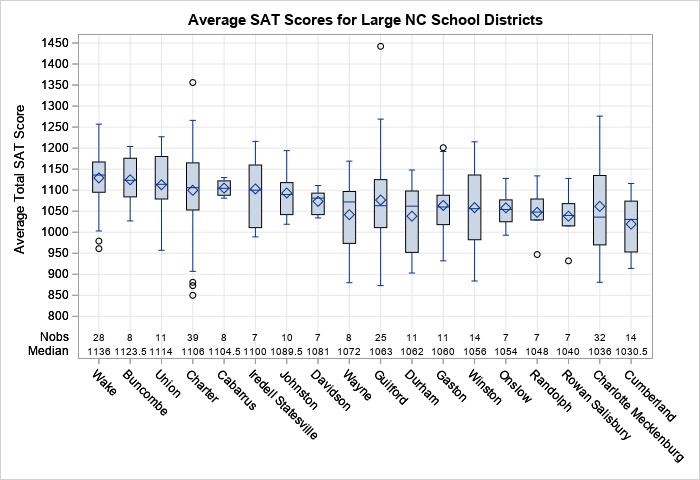The graph shows schematic box plots for 18 large school districts. The districts are sorted according to the median value of the schools' SAT scores. The INSETGROUP statement creates a table inside the graph. The table shows the number of schools in each district and gives the median score for the district. The INSETGROUP statement can display many other statistics such as the mean, standard deviation, minimum value, and maximum value for each district.

### Automatically create panels of box plots

One of the coolest features of PROC BOXPLOT is that it will automatically create a panel of box plots. It is difficult to visualize all 115 NC school districts in a single graph. The graph would be very wide (or tall) and the labels for the school districts would potentially collide. However, PROC BOXPLOT will split the display into a panel, which is extremely convenient if you plan to print the graphs on a piece of paper.

For example, the following call to PROC BOXPLOT results in box plots for 115 school districts. The procedure splits these box plots across a panel that contains five graphs and plots 23 box plots in each graph. Notice that I do not have to specify the number of graphs: the procedure uses the data to make an intelligent decision. To save space in this blog post, I omit three of the graphs and only show the first and last graphs:

```ods graphics / width=640px height=400px; title "Average SAT Scores for NC School Districts"; proc boxplot data=SATSortMerge; plot Total*DistrictAbbr / grid odstitle=title nohlabel boxstyle=schematicID vaxis=800 to 1450 by 50; run;```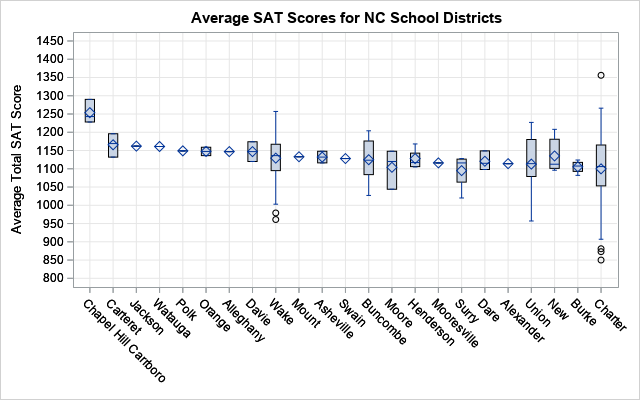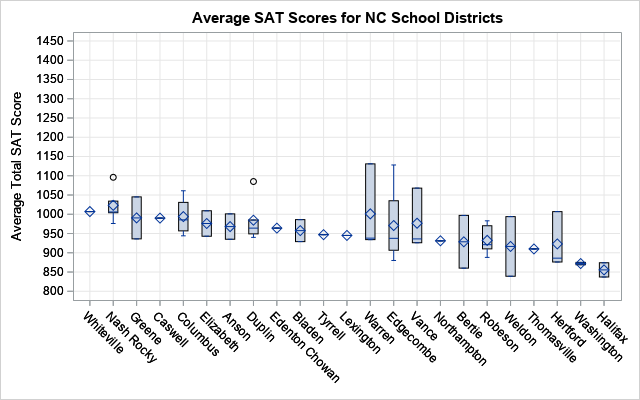Because the districts are ordered by the median SAT score, the first plot shows the school districts with high SAT scores and the last plot shows districts with lower SAT scores. Districts that have only one school are shown as a diamond (the mean value) with a line through it (the median value). Districts that have two or three schools are shown as a box without whiskers. For larger school districts, the box plots show a schematic representation of the distribution of the schools' SAT scores.

### Summary

In summary, PROC BOXPLOT has several useful features for plotting many box plots. This article shows that you can use the INSETGROUP statement to easily add a table of descriptive statistics to the graph. The procedure also automatically creates a panel of graphs so that you can more easily look at dozens or hundreds of box plots.

The post Use PROC BOXPLOT to display hundreds of box plots appeared first on The DO Loop.A previous article shows how to use a scatter plot to visualize the average SAT scores for all high schools in North Carolina. The schools are grouped by school districts and ranked according to the median value of the schools in the district. For the school districts that have many schools, the markers might overlap, which makes it difficult to visualize the distribution of scores. This is a general problem with using dot plots. An alternative visualization is to plot a box plot for each school district, which is described in today's article.

### Box plots are not for everyone

Box plots (also called box-and-whisker plots) are used by statisticians to provide a schematic visualization of the distribution of some quantity. The previous article was written for non-statisticians, so I did not include any box plots. To understand a box plot, the reader needs to know how to interpret the box and whiskers:

• The lower and upper portion of the box correspond to the 25th and 75th percentiles of the data, respectively
• The line in the middle of the box indicates the median score.
• A diamond marker indicates the mean score.
• The standard box plot extends the whiskers to observations that are within a distance D from the box, where D is 1.5 times the interquartile range. Other kinds of box plots are sometimes used. The SAS documentation explains the parts of a box plot.

Box plots require a certain level of comfort with statistical ideas. Nevertheless, for a statistical audience, box plots provide a compact way to compare dozens or hundreds of distributions.

### The BOXPLOT procedure: An alternative way to display many box plots

I almost always use the SGPLOT procedure to create box plots, but today I'm going to demonstrate the BOXPLOT procedure. The BOXPLOT procedure is from the days before ODS graphics, but it has several nice features, including the following:

1. When grouping box plots into categories, you can add headers, category dividers, and colors to help distinguish the categories. I demonstrated these so-called "nested box plots" in a previous blog post.
2. When you want to display statistics about each box plot as a table inside the graph, the BOXPLOT procedure provides a simple syntax. You can use PROC SGPLOT to add a statistical table to a box plot, but you need to pre-compute the statistics and merge the statistics and the data.
3. When you are plotting dozens or hundreds of box plots, the BOXPLOT procedure automatically splits the graph into a series of panels.

The second and third features are both useful for visualizing the SAT data for public high schools in NC.

### Add a statistical table to a graph that contains many box plots

You can use the INSETGROUP statement in PROC BOXPLOT to specify statistics that you want to display under each box plot. For example, the following syntax displays the number of high schools in each district and the median of the schools' SAT scores. The WHERE clause filters the data so that the graph shows only the largest school districts (those with seven or more high schools).

```ods graphics / width=700px height=480px; title "Average SAT Scores for Large NC School Districts"; proc boxplot data=SATSortMerge; where _FREQ_ >= 7; /* restrict to large school districts */ plot Total*DistrictAbbr / grid odstitle=title nohlabel boxstyle=schematicID vaxis=800 to 1450 by 50; insetgroup Q2 N; run;```The graph shows schematic box plots for 18 large school districts. The districts are sorted according to the median value of the schools' SAT scores. The INSETGROUP statement creates a table inside the graph. The table shows the number of schools in each district and gives the median score for the district. The INSETGROUP statement can display many other statistics such as the mean, standard deviation, minimum value, and maximum value for each district.

### Automatically create panels of box plots

One of the coolest features of PROC BOXPLOT is that it will automatically create a panel of box plots. It is difficult to visualize all 115 NC school districts in a single graph. The graph would be very wide (or tall) and the labels for the school districts would potentially collide. However, PROC BOXPLOT will split the display into a panel, which is extremely convenient if you plan to print the graphs on a piece of paper.

For example, the following call to PROC BOXPLOT results in box plots for 115 school districts. The procedure splits these box plots across a panel that contains five graphs and plots 23 box plots in each graph. Notice that I do not have to specify the number of graphs: the procedure uses the data to make an intelligent decision. To save space in this blog post, I omit three of the graphs and only show the first and last graphs:

```ods graphics / width=640px height=400px; title "Average SAT Scores for NC School Districts"; proc boxplot data=SATSortMerge; plot Total*DistrictAbbr / grid odstitle=title nohlabel boxstyle=schematicID vaxis=800 to 1450 by 50; run;```Because the districts are ordered by the median SAT score, the first plot shows the school districts with high SAT scores and the last plot shows districts with lower SAT scores. Districts that have only one school are shown as a diamond (the mean value) with a line through it (the median value). Districts that have two or three schools are shown as a box without whiskers. For larger school districts, the box plots show a schematic representation of the distribution of the schools' SAT scores.

### Summary

In summary, PROC BOXPLOT has several useful features for plotting many box plots. This article shows that you can use the INSETGROUP statement to easily add a table of descriptive statistics to the graph. The procedure also automatically creates a panel of graphs so that you can more easily look at dozens or hundreds of box plots.

The post Use PROC BOXPLOT to display hundreds of box plots appeared first on The DO Loop.Standardized tests like the SAT and ACT can cause stress for both high school students and their parents, but according to a Wall Street Journal article, the SAT and ACT "provide an invaluable measure of how students are likely to perform in college and beyond." Naturally, students wonder how their individual scores compare with others at their high school, their school district, or their state. I addressed some of those questions in a previous article that visualizes national ACT scores among college-bound students.

The average test scores at a school (or in a school system) are also scrutinized. Administrators and state legislators look at standardized scores to help assess the effectiveness of school districts, principals, and teachers. Parents might use scores to help them decide whether to send their child to the local public school, a charter school, or a private school.

A recent article in the Raleigh News and Observer links to a website of the NC Department of Public Instruction that contains the full set of SAT scores for all 496 public schools in NC. The table is great if you want to find the scores for a particular school, but is not very illuminating if you want an overview of how SAT scores vary at schools across the state. Thus, I decided to visualize the data for all NC schools by using SAS.

The data are only for public schools. You can download the data and the SAS program that I used to create the graphs in this article.

### The distribution of total SAT scores

The SAT score contains two components, a score on a math test and a score on a reading and writing test (sometimes referred to as the "English" score). Colleges look at the component scores and the sum of the scores (the "total" score). The following histogram shows the distribution of the average total SAT score for schools in North Carolina: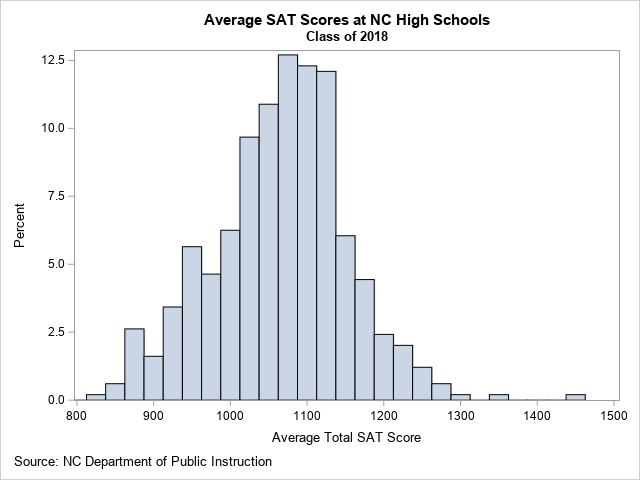From this graph, you can determine several facts about the data:

1. For most NC schools, the average SAT score is about 1100.
2. About 73% of NC schools have an average SAT score between 1000 and 1200.
3. There are a few schools that have much higher scores than the others. Those schools are Early College At Guilford (Total=1442), Raleigh Charter High School (Total=1356), and East Chapel Hill High (Total=1290).

### Visualize the SAT math and English scores

If you want to compare the distributions of the math and English SAT scores, you can create a comparative histogram, as shown below: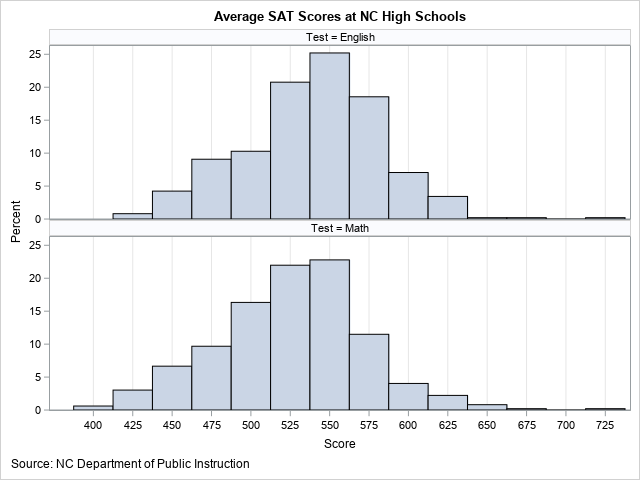In the comparative histogram, the average English scores for schools are shown in the top row; the math scores are in the bottom row. The English scores are generally higher, with a median value of 543. The median math score is about 15 points lower, with a value of 528.

Of course, the measurements in these two histograms are not independent. In reality, the math and English scores are paired, since every student takes both the math and English tests. Therefore, it makes sense to plot the joint distribution of the scores in a scatter plot, as follows: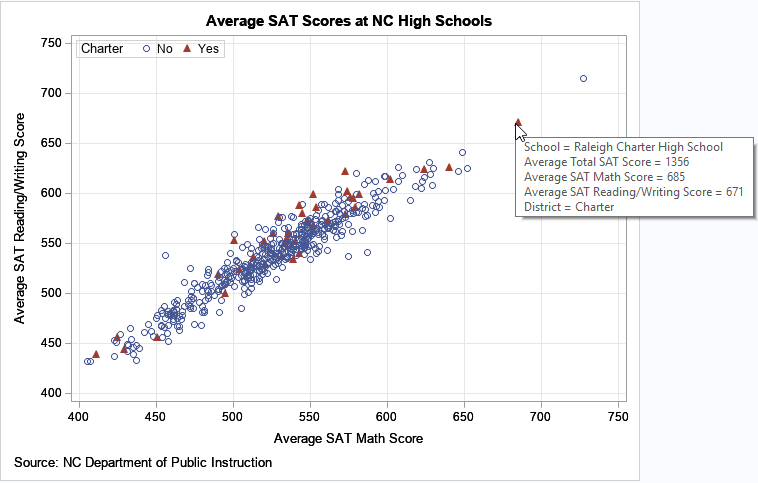In this plot, each school is represented by a marker. The math and English scores determine the placement of the marker. You can see that the math and English scores are highly correlated and linearly related. Schools with high (respectively, low) English scores tend to have high (respectively, low) math scores.

I used SAS to add tool tips to the graph. When I hover the cursor over a marker, the graph display information about the school and the average test scores. That technique makes it easy to identify the outliers. For example, the image above shows the tool-tip information for the Raleigh Charter High School, which has exceptionally high SAT scores.

And speaking of charter schools, legislators and school boards sometimes discuss the merits and disadvantages of using public tax dollars to establish and run charter schools. I have used red triangles to indicate the 39 charter schools in NC, which represent about 8% of the total schools. Overall, the distribution of SAT scores among the charter schools appears to be similar to the non-charter schools. I also created a comparative histogram of the charter/non-charter schools (not shown), which shows that the overall distribution is similar.

### SAT scores for all NC public high schools

The previous graphs show the distribution of SAT scores for all public NC high schools, but do not enable you to easily compare different school districts. One way to compare school districts is to rank them according to the median scores for the schools within the district. You can create a graph that shows the school scores for each district. Because there are 115 school districts in NC, this graph will be very tall or wide. Nevertheless, it can be useful to rank the school districts and simultaneously see the distribution of scores for each school. The following plot displays the top 75 school districts, which collectively contain 385 high schools: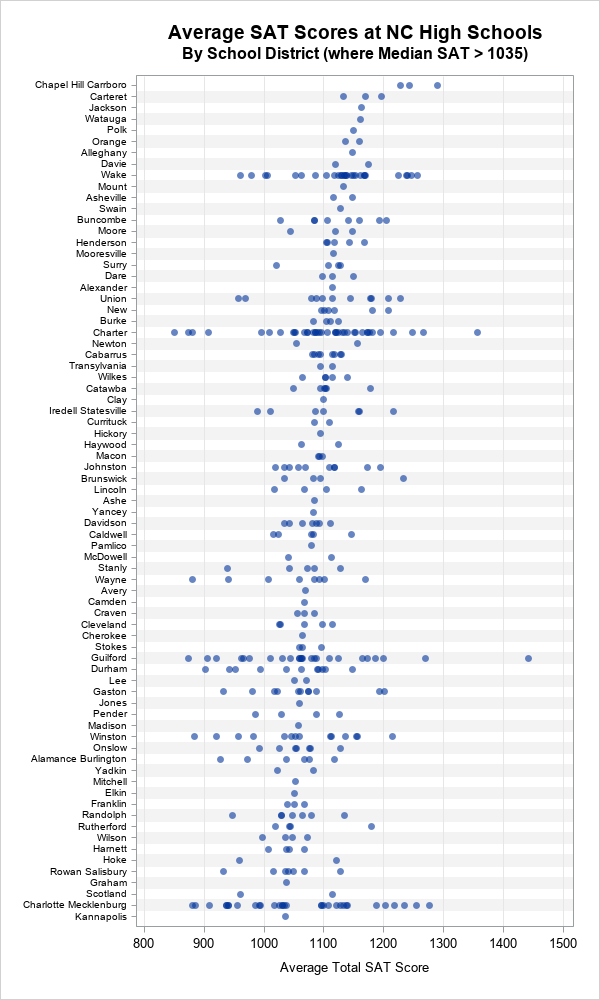The top school district is Chapel-Hill/Carrboro, which contains three high-performing high schools. The next few school districts are also small, having three or fewer high schools. Wake County, the second-largest school district in the state, is ninth in terms of the median SAT scores, but you can see considerable variation among the schools in the district. Other large school districts (Charter schools, Guilford, and Charlotte/Mecklenburg), show similar variation in scores.

### Summary

By using data visualization, you can better understand the distribution of SAT scores across public high schools in NC. The graphs in this article enable you to see that there are several high-performing schools, lots of schools in the middle, and a few low-performing schools. Similarly, you can compare the test scores by school districts. The school-district graph enables you to see the variation of scores of schools within a district. Lastly, when you create these graphs in SAS, you can add tool tips that help you identify the individual schools within each school district.

In my next article, I will describe an alternative visualization that displays a box plot for each school district.

The post Visualize SAT scores in North Carolina appeared first on The DO Loop.Box plots are a great way to compare the distributions of several subpopulations of your data. For example, box plots are often used in clinical studies to visualize the response of patients in various cohorts. This article describes three techniques to visualize responses when the cohorts have a nested or hierarchical structure, such as experimental treatments nested inside of clinics. The techniques are:

• Use PROC SGPLOT (or PROC SGPANEL): Use the VBOX statement to visualize the nested structure. You can use the CATEGORY= option to specify the "outer" variable and the GROUP= option to specify the "inner" (nested) variable.
• Use PROC GLM: For a model that has exactly two categorical variables, one nested in the other, PROC GLM automatically creates a nested box plot.
• Use PROC BOXPLOT: You can use PROC BOXPLOT to create a nested box plot. The procedure supports several options that can enhance the visualization.

### An example of nested data: Leaves on plants

Did you know that turnip greens are an excellent source of calcium? The following example is from the PROC NESTED documentation and is based on data analyzed in Snedecor and Cochran (Statistical Methods, 6th ed., 1967, p. 286). The original data is from an experiment in which four random turnip green plants are selected, then three leaves are randomly selected on each plant. From each leaf, two 100-mg samples were selected and used to determine the amount of calcium. (The units for calcium are percentage of dry weight.) Because the box plot for a two-element sample is trivial, I made up four additional fake measurements of calcium for each leaf, as shown in the following DATA step:

```/* PROC NESTED example. First two measurements are real data. The last four are fake. */ data Turnip; do Plant=1 to 4; do Leaf=1 to 3; do Sample=1 to 6; input Calcium @@; output; end; end; end; /* |--REAL--| |----- FAKE ------| */ datalines; 3.28 3.09 3.26 3.19 3.27 3.01 3.52 3.48 3.53 3.47 3.50 3.49 2.88 2.80 2.98 2.70 2.28 2.81 2.46 2.44 2.58 2.30 2.61 2.48 1.87 1.92 1.97 1.90 1.86 1.90 2.19 2.19 2.21 2.17 2.23 2.19 2.77 2.66 2.79 2.63 2.72 2.69 3.74 3.44 3.75 3.45 3.73 3.34 2.55 2.55 2.59 2.51 2.49 2.61 3.78 3.87 3.79 3.81 3.88 3.76 4.07 4.12 4.23 4.27 4.01 4.08 3.31 3.31 3.30 3.34 3.33 3.30 ;   title 'Calcium Concentration in Turnip Leaves'; title2 'Leaves Nested Within Plants'; footnote J=L 'Based on Snedecor and Cochran (1967, p. 286)'; proc sgplot data=Turnip; vbox Calcium / Group=Leaf category=Plant; xaxis discreteorder=data; run;```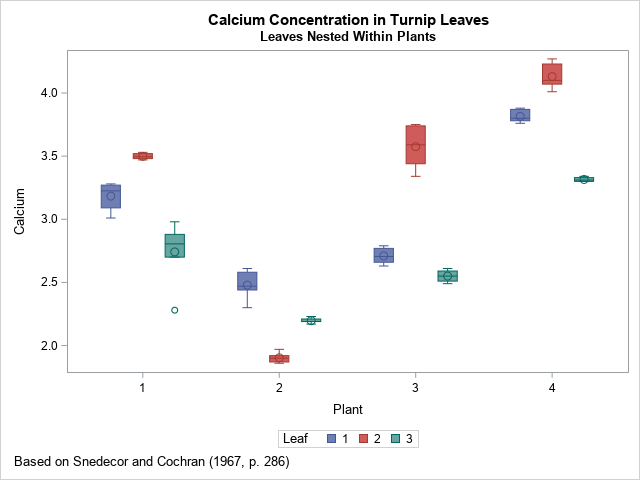This simple visualization uses the VBOX statement in PROC SGPLOT. As I explained previously, you can use the CATEGORY= and GROUP= options to display the distribution of calcium for the joint levels of the two categorical variables. The CATEGORY= option specifies the horizontal variable; the GROUP= option specifies the levels of a second variable. In this case, the levels of the LEAF variable are nested inside the levels of the PLANT variable. The result is shown. Colors are used to identify the level of the GROUP= variable.

If there were additional levels of nesting (for examples, multiple farms), you could use the SGPANEL procedure and include the additional variables on the PANELBY statement.

### Visualize a nested ANOVA model

You can improve the previous graph by visually dividing the leaves in one plant from the leaves in another. You can use PROC GLM to automatically display the divisions when you analyze a model for which the explanatory categorical variables are nested. The following call to PROC GLM specifies a nested mode. The "NestPlot" graph is created automatically when you use ODS GRAPHICS:

```ods graphics on; proc glm data=Turnip; class Plant Leaf; model Calcium = Leaf(Plant); /* Leaf nested in Plant */ quit;```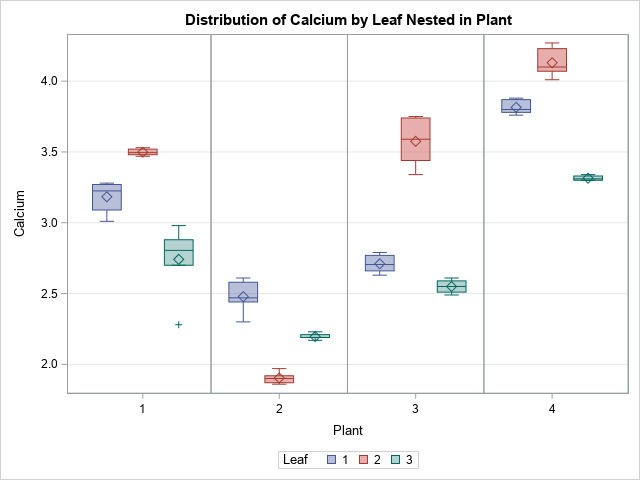As you can see, the graph is the same except that it contains vertical lines that divide one plant from another. I like this version of the graph better.

### Box plots for independent units

If you think about it, the color in the previous plot is not necessary. You might even consider it misleading because the leaf values are unrelated across plants. "Leaf 1" for "Plant 1" has no relationship to "Leaf 1" for the other plants. To emphasize that fact, you could relabel the leaves by using the values 1 through 12, where leaves 1–3 are from Plant=1, leaves 4–6 are from Plant=2, and so forth.

Labeling the leaves that way is necessary if you want to use to BOXPLOT procedure to visualize the data. The BOXPLOT procedure supports nested categories and the syntax is similar to the GLM syntax:

```data Turnip2; set Turnip; LeafID = Leaf + (Plant-1)*3; /* label samples 1, 2, 3, ..., 12 */ run;   proc boxplot data=Turnip2; plot Calcium*LeafID(Plant) / odstitle=title odstitle2=title2 odsfootnote=footnote; run;```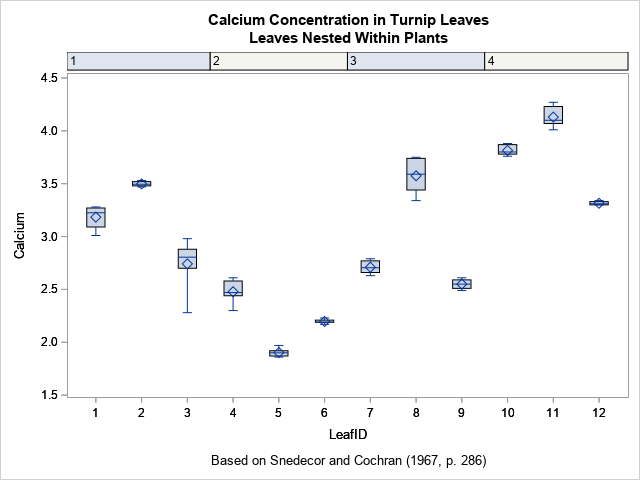The output from the BOXPLOT procedure uses column headers to indicate the plants. This is similar to the visualization that I used to label categories for tropical storms and hurricanes.

You can use three features of SAS/STAT 15.1 (SAS 9.4M6) to add vertical reference lines, to color the background, and to center the plant labels in the column headers. The options are the BLOCKREF option, the BLOCKREFFILL option, and the BLOCKVALUEPOS= option, respectively.

```/* Options for extending the column headers into the plot region. These options require SAS/STAT 15.1 */ proc boxplot data=Turnip2; plot Calcium*LeafID(Plant) / odstitle=title odstitle2=title2 odsfootnote=footnote blockref blockreffill blockvaluepos=center; run;```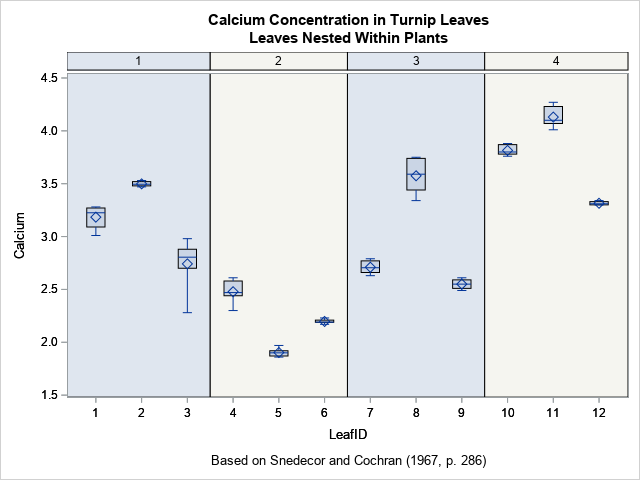I like this graph because it uses colors sparingly and does not require a legend. Also, if the number of samples is large (such as 30 or 50), PROC BOXPLOT will automatically produce a series of graphs, each displaying a portion of the data.

In summary, this article shows three ways to create box plots of responses for nested categorical data. The simplest is to use the VBOX statement in PROC SGPLOT, although if there are additional categorical variables in the model, you can create a lattice of box plots by using the PANELBY statement in PROC SGPANEL. The GLM procedure enables you to perform an ANOVA analysis for the data and also create a visualization. The BOXPLOT procedure provides nice headers and (in SAS/STAT 15.1) colored strips for each level of the "outer" categories.

The post 3 ways to create nested box plots in SAS appeared first on The DO Loop.Box plots are a great way to compare the distributions of several subpopulations of your data. For example, box plots are often used in clinical studies to visualize the response of patients in various cohorts. This article describes three techniques to visualize responses when the cohorts have a nested or hierarchical structure, such as experimental treatments nested inside of clinics. The techniques are:

• Use PROC SGPLOT (or PROC SGPANEL): Use the VBOX statement to visualize the nested structure. You can use the CATEGORY= option to specify the "outer" variable and the GROUP= option to specify the "inner" (nested) variable.
• Use PROC GLM: For a model that has exactly two categorical variables, one nested in the other, PROC GLM automatically creates a nested box plot.
• Use PROC BOXPLOT: You can use PROC BOXPLOT to create a nested box plot. The procedure supports several options that can enhance the visualization.

### An example of nested data: Leaves on plants

Did you know that turnip greens are an excellent source of calcium? The following example is from the PROC NESTED documentation and is based on data analyzed in Snedecor and Cochran (Statistical Methods, 6th ed., 1967, p. 286). The original data is from an experiment in which four random turnip green plants are selected, then three leaves are randomly selected on each plant. From each leaf, two 100-mg samples were selected and used to determine the amount of calcium. (The units for calcium are percentage of dry weight.) Because the box plot for a two-element sample is trivial, I made up four additional fake measurements of calcium for each leaf, as shown in the following DATA step:

```/* PROC NESTED example. First two measurements are real data. The last four are fake. */ data Turnip; do Plant=1 to 4; do Leaf=1 to 3; do Sample=1 to 6; input Calcium @@; output; end; end; end; /* |--REAL--| |----- FAKE ------| */ datalines; 3.28 3.09 3.26 3.19 3.27 3.01 3.52 3.48 3.53 3.47 3.50 3.49 2.88 2.80 2.98 2.70 2.28 2.81 2.46 2.44 2.58 2.30 2.61 2.48 1.87 1.92 1.97 1.90 1.86 1.90 2.19 2.19 2.21 2.17 2.23 2.19 2.77 2.66 2.79 2.63 2.72 2.69 3.74 3.44 3.75 3.45 3.73 3.34 2.55 2.55 2.59 2.51 2.49 2.61 3.78 3.87 3.79 3.81 3.88 3.76 4.07 4.12 4.23 4.27 4.01 4.08 3.31 3.31 3.30 3.34 3.33 3.30 ;   title 'Calcium Concentration in Turnip Leaves'; title2 'Leaves Nested Within Plants'; footnote J=L 'Based on Snedecor and Cochran (1967, p. 286)'; proc sgplot data=Turnip; vbox Calcium / Group=Leaf category=Plant; xaxis discreteorder=data; run;```This simple visualization uses the VBOX statement in PROC SGPLOT. As I explained previously, you can use the CATEGORY= and GROUP= options to display the distribution of calcium for the joint levels of the two categorical variables. The CATEGORY= option specifies the horizontal variable; the GROUP= option specifies the levels of a second variable. In this case, the levels of the LEAF variable are nested inside the levels of the PLANT variable. The result is shown. Colors are used to identify the level of the GROUP= variable.

If there were additional levels of nesting (for examples, multiple farms), you could use the SGPANEL procedure and include the additional variables on the PANELBY statement.

### Visualize a nested ANOVA model

You can improve the previous graph by visually dividing the leaves in one plant from the leaves in another. You can use PROC GLM to automatically display the divisions when you analyze a model for which the explanatory categorical variables are nested. The following call to PROC GLM specifies a nested mode. The "NestPlot" graph is created automatically when you use ODS GRAPHICS:

```ods graphics on; proc glm data=Turnip; class Plant Leaf; model Calcium = Leaf(Plant); /* Leaf nested in Plant */ quit;```As you can see, the graph is the same except that it contains vertical lines that divide one plant from another. I like this version of the graph better.

### Box plots for independent units

If you think about it, the color in the previous plot is not necessary. You might even consider it misleading because the leaf values are unrelated across plants. "Leaf 1" for "Plant 1" has no relationship to "Leaf 1" for the other plants. To emphasize that fact, you could relabel the leaves by using the values 1 through 12, where leaves 1–3 are from Plant=1, leaves 4–6 are from Plant=2, and so forth.

Labeling the leaves that way is necessary if you want to use to BOXPLOT procedure to visualize the data. The BOXPLOT procedure supports nested categories and the syntax is similar to the GLM syntax:

```data Turnip2; set Turnip; LeafID = Leaf + (Plant-1)*3; /* label samples 1, 2, 3, ..., 12 */ run;   proc boxplot data=Turnip2; plot Calcium*LeafID(Plant) / odstitle=title odstitle2=title2 odsfootnote=footnote; run;```The output from the BOXPLOT procedure uses column headers to indicate the plants. This is similar to the visualization that I used to label categories for tropical storms and hurricanes.

You can use three features of SAS/STAT 15.1 (SAS 9.4M6) to add vertical reference lines, to color the background, and to center the plant labels in the column headers. The options are the BLOCKREF option, the BLOCKREFFILL option, and the BLOCKVALUEPOS= option, respectively.

```/* Options for extending the column headers into the plot region. These options require SAS/STAT 15.1 */ proc boxplot data=Turnip2; plot Calcium*LeafID(Plant) / odstitle=title odstitle2=title2 odsfootnote=footnote blockref blockreffill blockvaluepos=center; run;```I like this graph because it uses colors sparingly and does not require a legend. Also, if the number of samples is large (such as 30 or 50), PROC BOXPLOT will automatically produce a series of graphs, each displaying a portion of the data.

In summary, this article shows three ways to create box plots of responses for nested categorical data. The simplest is to use the VBOX statement in PROC SGPLOT, although if there are additional categorical variables in the model, you can create a lattice of box plots by using the PANELBY statement in PROC SGPANEL. The GLM procedure enables you to perform an ANOVA analysis for the data and also create a visualization. The BOXPLOT procedure provides nice headers and (in SAS/STAT 15.1) colored strips for each level of the "outer" categories.

The post 3 ways to create nested box plots in SAS appeared first on The DO Loop.When you overlay two series in PROC SGPLOT, you can either plot both series on the same axis or you can assign one series to the main axis (Y) and another to a secondary axis (Y2). If you use the Y and Y2 axes, they are scaled independently by default, which is usually what you want. However, if the measurements for the two series are linearly related to each other, then you might want to specify the tick values for the Y2 axis so that they align with the corresponding tick marks for the Y axis. This article shows how to align the Y and Y2 axes in PROC SGPLOT in SAS for two common situations.

### Different scales for one set of measurements

The simplest situation is a single set of data that you want to display in two different units. For example, you might use one axis to display the data in imperial units (pounds, gallons, degrees Fahrenheit, etc.) and the other axis to display the data in metric units (kilograms, liters, degrees Celsius, etc.).

To plot the data, define one variable for each unit. For example, the Sashelp.Class data records the weight for 19 students in pounds. The following DATA view creates a new variable that records the same data in kilograms. The subsequent call to PROC SGPLOT plots the pounds on the Y axis (left axis) and the kilograms on the Y2 axis (right axis). However, as you will see, there is a problem with the default scaling of the two axes:

```data PoundsKilos / view=PoundsKilos; set Sashelp.Class(rename=(Weight=Pounds)); Kilograms = 0.453592 * Pounds; /* convert pounds to kilos */ run;   title "Independent Axes"; title2 "Markers Do Not Align Correctly!"; /* the tick marks on each axis are independent */ proc sgplot data=PoundsKilos; scatter x=Height y=Pounds; scatter x=Height y=Kilograms / Y2Axis; run;```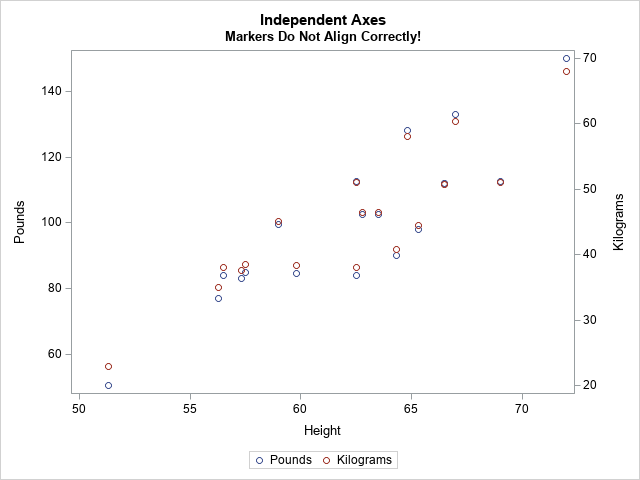The markers for the kilogram measurements should exactly overlap the markers for pounds, but they don't. The Y and Y2 axes are independently scaled because PROC SGPLOT does not know that pounds and kilograms are linearly related. The SGPLOT procedure displays each variable by using a range of round numbers (multiples of 10 or 20). The range for the Y2 axis is [20, 70] kilograms, which corresponds to a range of [44.1, 154.3] pounds. However, the range for the Y axis is approximately [50, 150] pounds. Because the axes display different ranges, the markers do not overlap.

To improve this graph, use the VALUES= and VALUESDISPLAY= options on the YAXIS statement (or Y2AXIS statement) to force the ticks marks on one axis to align with the corresponding tick marks on the other axis. In the following DATA step, I use the kilogram scale as the standard and compute the corresponding pounds.

```data Ticks; do Kilograms = 20 to 70 by 10; /* for each Y2 tick */ Pounds = Kilograms / 0.453592; /* convert kilos to pounds */ Approx = round(Pounds, 0.1); /* use rounded values to display tick values */ output; end; run; proc print; run;```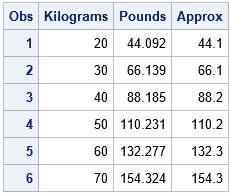You can use the Pounds column in the table to set the VALUES= list on the YAXIS statement. You can use the Approx column to set the VALUESDISPLAY= list, as follows:

```/* align tick marks on each axis */ title "Both Axes Use the Same Scale"; proc sgplot data=PoundsKilos noautolegend; scatter x=Height y=Pounds; /* Make sure the plots overlay exactly! Then you can set SIZE=0 */ scatter x=Height y=Kilograms / markerattrs=(size=0) Y2Axis; yaxis grid values=(44.092 66.139 88.185 110.231 132.277 154.324) valuesdisplay=('44.1' '66.1' '88.2' '110.2' '132.3' '154.3'); run;```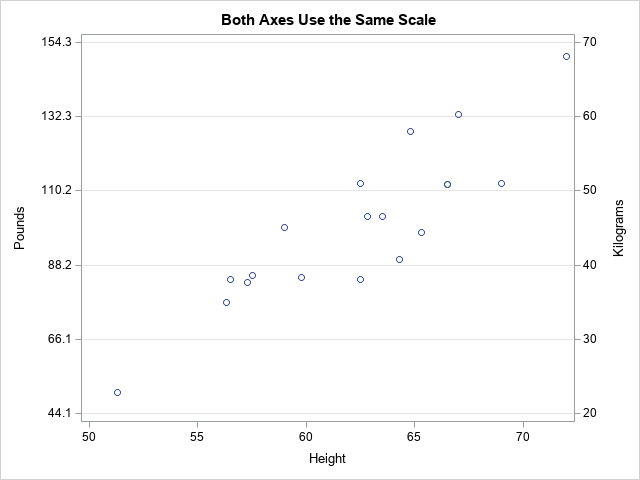Success! The markers for the two variables align exactly. After verifying that they align, you can use the MARKERATTRS=(SIZE=0) option to suppress the display of one of the markers.

Notice that the Y axis (pounds) no longer displays "nice numbers" because I put the tick marks at the same vertical heights on both axes. A different way to solve the misalignment problem is to use the MIN=, MAX=, THRESHOLDMIN=, and THRESHOLDMAX= options on both axes. This will enable both axes to use "nice numbers" while still aligning the data. If you want to try this approach, here are the YAXIS and Y2AXIS statements:

``` /* set the axes ranges to coresponding values */ yaxis grid thresholdmin=0 thresholdmax=0 min=44.1 max=154.3; y2axis grid thresholdmin=0 thresholdmax=0 min=20 max=70;```

### Different scales for different measurements

Another situation that requires two Y axes is the case of two series that use different units. For example, you might want to plot the revenue for a US company (in dollars) and the revenue for a Japanese company (in yen) for a certain time period. You can use the conversion rate between yen and dollars to align the values on the axes. Of course, the conversion from Japanese yen to the US dollars changes each day, but you can use an average conversion rate to set the correspondence between the axes.

This situation also occurs when two devices use different methods to measure the same quantity. The following example shows measurements for a patient who receives a certain treatment. The quantity of a substance in the patient's blood is measured at baseline and for every hour thereafter. The quantity is measured in two ways: by using a traditional blood test and by using a new noninvasive device that measures electrical impedance. The following statements define and plot the data. The two axes are scaled by using the default method:

```data BloodTest1; label t="Hours after Medication" x="micrograms per deciliter" y="kiloOhms"; input x y @@; t = _N_ - 1; datalines; 169.0 45.5 130.8 33.4 109.0 23.8 94.1 19.8 86.3 20.4 78.4 18.7 76.1 16.1 72.2 16.7 70.0 11.9 69.8 14.6 69.5 10.6 68.7 12.7 67.3 16.9 ;   title "Overlay Measurements for Two Medical Devices"; title2 "Default Scaling"; proc sgplot data=BloodTest1; series x=t y=x / markers legendlabel="Standard Lab Value"; series x=t y=y / markers Y2Axis legendlabel="New Device"; xaxis values=(0 to 12 by 2); yaxis grid label="micrograms per deciliter"; y2axis grid label="kiloOhms"; run;```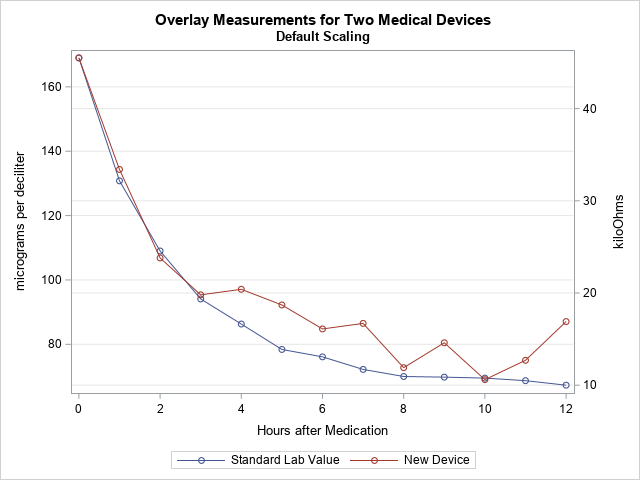In this graph, the Y axes are scaled independently. However, the company that manufactures the device used Deming regression to establish that the measurements from the two devices are linearly related by the equation Y = –10.56415 + 0.354463*X, where X is the measurement from the blood test. You can use this linear equation to set the scales for the two axes.

The following DATA step uses the Deming regression estimates to convert the tick marks on the Y axis into values for the Y2 axis. (Click here for the PROC PRINT output.) The call to PROC SGPLOT creates a graph in which the Y2 axis is aligned with the Y axis according to the Deming regression estimates.

```data Ticks; do Y1 = 60 to 160 by 20; /* use Deming regression to find one set of ticks in terms of the other */ Y2 = -10.56415 + 0.354463 * Y1; /* kiloOhms as a function of micrograms/dL */ Approx = round(Y2, 0.1); output; end; run;   proc print; run;   title "Align Y Axes for Different Series"; title2 "Measurements are Linearly Related"; proc sgplot data=BloodTest1; series x=t y=x / markers legendlabel="Standard Lab Value"; series x=t y=y / markers Y2Axis legendlabel="New Device"; xaxis values=(0 to 12 by 2); yaxis grid label="micrograms per deciliter" offsetmax=0.1 values=(60 to 160 by 20); /* the same offsets must be used in both YAXIS and Y2AXIS stmts */ y2axis grid label="kiloOhms" offsetmax=0.1 values=(10.7036 17.7929 24.8822 31.9714 39.0607 46.1499) valuesdisplay=('10.7' '17.8' '24.9' '32.0' '39.1' '46.1'); run;```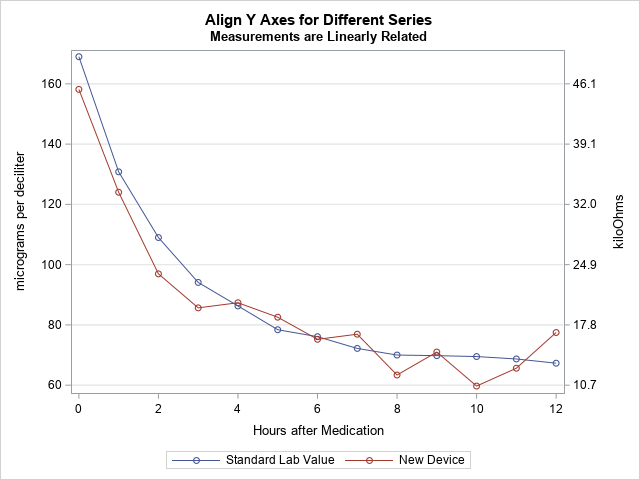In this new graph, the measurements are displayed on compatible scales and the reference lines connect round numbers on one axis to the corresponding values on the other axis.

The post How to align the Y and Y2 axes in PROC SGPLOT appeared first on The DO Loop.Numbers don't lie, but sometimes they don't reveal the full story. Last week I wrote about the most popular articles from The DO Loop in 2018. The popular articles are inevitably about elementary topics in SAS programming or statistics because those topics have broad appeal. However, I also write about advanced topics, which are less popular but fill an important niche in the SAS community. Not everyone needs to know how to fit a Pareto distribution in SAS or how to compute distance-based measures of correlation in SAS. Nevertheless, these topics are interesting to think about.

I believe that learning should not stop when we leave school. If you, too, are a lifelong learner, the following topics deserve a second look. I've included articles from four different categories.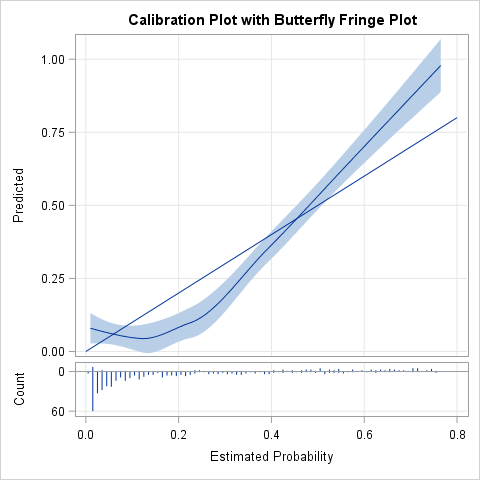### Random numbers and resampling methods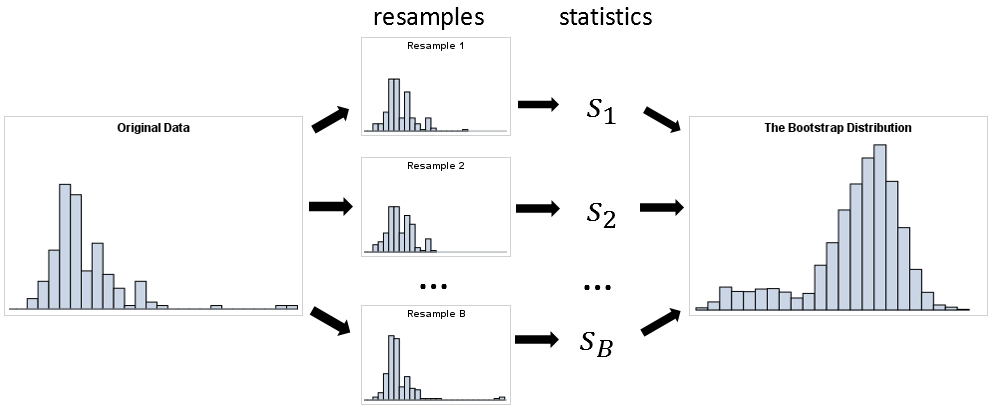### Optimization

These articles are technical but provide tips and techniques that you might find useful. Choose a few topics that are unfamiliar and teach yourself something new in this New Year!

Do you have a favorite article from 2018 that I did not include on the list? Share it in a comment!

The post 10 posts from 2018 that deserve a second look appeared first on The DO Loop.Last year, I wrote more than 100 posts for The DO Loop blog. Of these, the most popular articles were about data visualization, SAS programming tips, and statistical data analysis. Here are the most popular articles from 2018 in each category.

### Data Visualization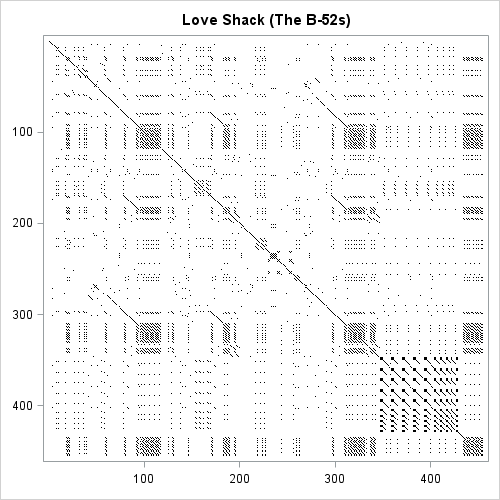### Statistics and Data Analysis

I write this blog because I love to learn new things and share what I know with others. If you want to learn something new, read (or re-read!) these popular articles from 2018. Then share this page with one of your colleagues. Happy New Year! I hope we both have many opportunities to learn and share in 2019!

The post Top posts from <em>The DO Loop</em> in 2018 appeared first on The DO Loop.I regularly see questions on a SAS discussion forum about how to visualize the predicted values for a mixed model that has at least one continuous variable, a categorical variable, and possibly an interaction term. SAS procedures such as GLM, GENMOD, and LOGISTIC can automatically produce plots of the predicted values versus the explanatory variables. These are called "fit plots" or "interaction plots" or "sliced fit plots." Although PROC MIXED does not automatically produce a "fit plot" for a mixed model, you can use the output from the procedure to construct a fit plot. In fact, two graphs are possible: one that incorporates the random effects for each subject in the predicted values and another that does not.

### Use PROC PLM to visualize the fixed-effect model

Because the MIXED (and GLIMMIX) procedure supports the STORE statement, you can write the model to an item store and then use the EFFECTPLOT statement in PROC PLM to visualize the predicted values. The resulting graph visualizes the fixed effects. The random effects are essentially "averaged out" or shown at their expected value, which is zero.

As an example, consider the following repeated measures example from the PROC MIXED documentation. The data are measurements for 11 girls and 16 boys recorded when the children were 8, 10, 12, and 14 years old. According to Pothoff and Roy (1964), "Each measurement is the distance, in millimeters, from the centre of the pituitary to the pteryomaxillary fissure. The reason why there is an occasional instance where this distance decreases with age is that the distance represents the relative position of two points." You can use a spaghetti plot to visualize the raw data:

```data pr; input Person Gender \$ y1 y2 y3 y4; y=y1; Age=8; output; y=y2; Age=10; output; y=y3; Age=12; output; y=y4; Age=14; output; drop y1-y4; label y="Relative Distance (mm)"; datalines; 1 F 21.0 20.0 21.5 23.0 2 F 21.0 21.5 24.0 25.5 3 F 20.5 24.0 24.5 26.0 4 F 23.5 24.5 25.0 26.5 5 F 21.5 23.0 22.5 23.5 6 F 20.0 21.0 21.0 22.5 7 F 21.5 22.5 23.0 25.0 8 F 23.0 23.0 23.5 24.0 9 F 20.0 21.0 22.0 21.5 10 F 16.5 19.0 19.0 19.5 11 F 24.5 25.0 28.0 28.0 12 M 26.0 25.0 29.0 31.0 13 M 21.5 22.5 23.0 26.5 14 M 23.0 22.5 24.0 27.5 15 M 25.5 27.5 26.5 27.0 16 M 20.0 23.5 22.5 26.0 17 M 24.5 25.5 27.0 28.5 18 M 22.0 22.0 24.5 26.5 19 M 24.0 21.5 24.5 25.5 20 M 23.0 20.5 31.0 26.0 21 M 27.5 28.0 31.0 31.5 22 M 23.0 23.0 23.5 25.0 23 M 21.5 23.5 24.0 28.0 24 M 17.0 24.5 26.0 29.5 25 M 22.5 25.5 25.5 26.0 26 M 23.0 24.5 26.0 30.0 27 M 22.0 21.5 23.5 25.0 ;   /* for information about the LEGENDITEM statement, see https://blogs.sas.com/content/iml/2018/02/12/merged-legends.html */ title "Relative Distance Between Features"; proc sgplot data=pr; series x=Age y=Y / group=Person groupLC=Gender curvelabel; /* LEGENDITEM is a SAS 9.4M5 feature. Delete the following statements in older versions of SAS */ legenditem type=line name="F" / label="Girls" lineattrs=GraphData1; legenditem type=line name="M" / label="Boys" lineattrs=GraphData2(pattern=dash); keylegend "M" "F"; run;```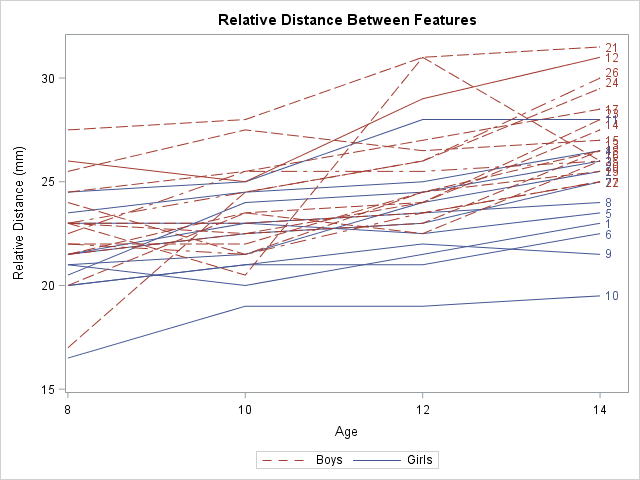Notice that I used the LEGENDITEM statement to customize the legend. The LEGENDITEM statement is a SAS 9.4M5 feature. You can delete the LEGENDITEM and KEYLEGEND statements to obtain the default legend.

One of the stated goals of the study is to model the average growth rate of the measurement for boys and for girls. You can see from the spaghetti plot that growth rate appears to be linear and that boys tend to have larger measurements than girls of the same age. However, it is not clear whether the rate (the slope of the average line) is the same for each gender or is significantly different.

The documentation example describes several ways to model the variance structure for the repeated measures. One choice is the AR(1) structure. The following statement uses the REPEATED statement to model the repeated measures. The STORE statement writes an item store that contains information about the model, which is used by PROC PLM to create effect plots:

```proc mixed data=pr method=ml; class Person Gender; model y = Gender Age Gender*Age / s; repeated / type=ar(1) sub=Person r; store out=MixedModel; /* create item store */ run;   proc plm restore=MixedModel; /* use item store to create fit plots */ effectplot fit(x=Age plotby=Gender); /* panel */ effectplot slicefit(x=Age sliceby=Gender); /* overlay */ *effectplot slicefit(x=Age sliceby=Person); /* ERROR: Person is not a fixed effect */ run;```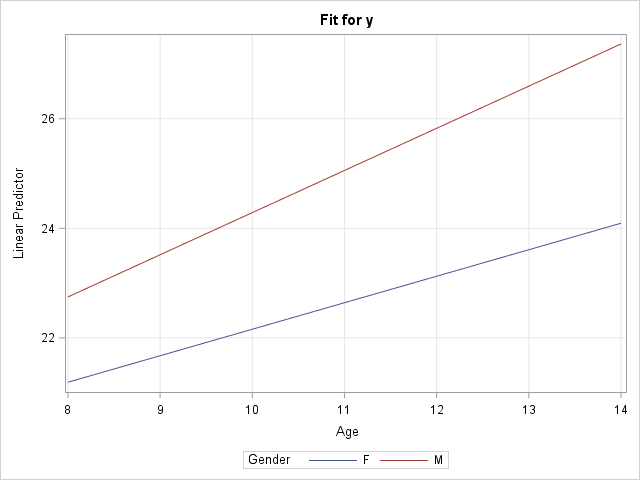The call to PROC PLM creates a panel of plots with confidence bands (not shown) and a graph that overlays the predicted values for males and females (shown). You can see a small difference in slopes between the two average growth curves, but the "Type 3 Tests of Fixed Effects" table from PROC MIXED (not shown) indicates that the difference is not statistically significant. The graph does not display the raw observations because PROC PLM does not have access to them. However, you can obtain a graph that overlays the observation by modifying the method in the next section.

Notice that this graph displays the model for boys and girls, but not for the individual subjects. In fact, I added a comment to the program that reminds you that it is an error to try to use the PERSON variable in the EFFECTPLOT statement because PERSON is not a fixed effect in the model. If you want to model to growth curves for individuals, see the next section.

### Use the OUTPRED= option visualize the random-coefficient model

The spaghetti plot seems to indicate that the growth curves for the individuals have the same slope but different intercepts. You can model this by using the RANDOM statement to add a random intercept effect to the model. The resulting graph "untangles" the spaghetti plot by plotting a line that best fits each individual's growth.

You can use the OUTPRED= option on the MODEL statement to create an output data set in which the predicted values incorporate the random intercept for each person:

```/* random coefficient model */ proc mixed data=pr method=ml; class Person Gender; model y = Gender Age Gender*Age / s outpred=Pred; random int / sub=Person; run;   /* BE SURE TO SORT by the X variable, before creating a SERIES plot. */ /* These data are already sorted, but the next line shows how to sort, if necessary */ proc sort data=Pred; by Gender Person Age; run;   title "Predicted Individual Growth Curves"; proc sgplot data=Pred; scatter x=Age y=Y / group=Gender; series x=Age y=Pred / group=Person GroupLC=Gender curvelabel; /* LEGENDITEM is a SAS 9.4M5 feature. Delete the following statements in older versions of SAS */ legenditem type=markerline name="F" / label="Girls" lineattrs=GraphData1 markerattrs=GraphData1; legenditem type=markerline name="M" / label="Boys" lineattrs=GraphData2(pattern=dash) markerattrs=GraphData2; keylegend "M" "F"; run;```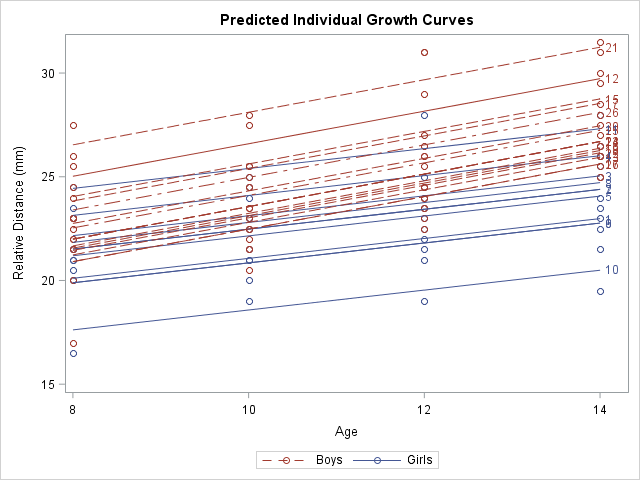This graph shows a smoothed version of the spaghetti plot. The graph enables you to see the variation in intercepts for the subjects but the slopes are determined by the gender of each individual. In statistical terms, the predicted values in this graph "incorporate the EBLUP values." The documentation contains the formula for the predicted values.

It is worth noting that the MODEL statement in PROC MIXED also supports an OUTPREDM= option. (The 'M' stands for 'marginal' model.) The OUTPREDM= option writes a data set that contains the predictions that do not "incorporate the EBLUP values", where EBLUP is an abbreviation for the "empirical best linear unbiased prediction." These are the same predicted values that you obtain from the STORE statement and PROC PLM. However, the OUTPREDM= data set gives you complete control over how you use the predicted values. For example, you can use the OUTPREDM= data set to add the original observations to the graph of the predicted values for boys and girls.

In summary, when you include a continuous covariate (like age) in a mixed model, there are two ways to visualize the "fit plot." You can use the STORE statement and PROC PLM to obtain a graph of the predictions from the "marginal model" that contains the fixed effects. (You can also use the OUTPREDM= option for this purpose.) Alternatively, if you are fitting a model that estimates random coefficients (intercepts or slopes), you can use the OUTPRED= option to write a data set that contains the predicted that incorporate the estimates.

The post Visualize a mixed model that has repeated measures or random coefficients appeared first on The DO Loop.